# Lions Weekly: January 26, 2022# A Note From Mr. Sandefur

Lion Family,

Good afternoon! We are quickly approaching our PTF’s Love Gala that will be an incredible night of fun and fellowship as we seek to honor those who have made an impact on TKA, as well as raise funds for our Legacy Fund. The Love Gala’s proceeds will be used to “love” our students, faculty, and facilities as they will cover projects aimed at improvements for each area of our campus life. Please consider joining us on Saturday, February 12th, as we “love” on The King’s Academy.

Speaking of events, TKA will be hosting the “Forged Parent Conference” on March 5th in Woody Auditorium. “Forged” will be a morning of biblical-based parenting seminars focused on encouraging parents that are raising students in a post-Christian society. We are blessed to have local ministers from our community coming to share on topics involving discipleship, maneuvering technology in today’s digital society, and student identity in an ever-changing environment. This event is free and will be a great opportunity to link arms with other parents as we focus on what the Bible says about the roles and responsibilities associated with raising children. I hope that you will make it a priority to join us from 9:00 – 12:00 on Saturday, March 5th for “Forged.”

In Christ Alone,

Jeremy Sandefur
President

 table div table+table+table+table div table{width:100%;padding:0}table div table+table+table+table div table img{width:96.23%;padding:0;float:none}table div table+table+table+table div table td{width:100%;padding:0 1.88% 18px}/* styles */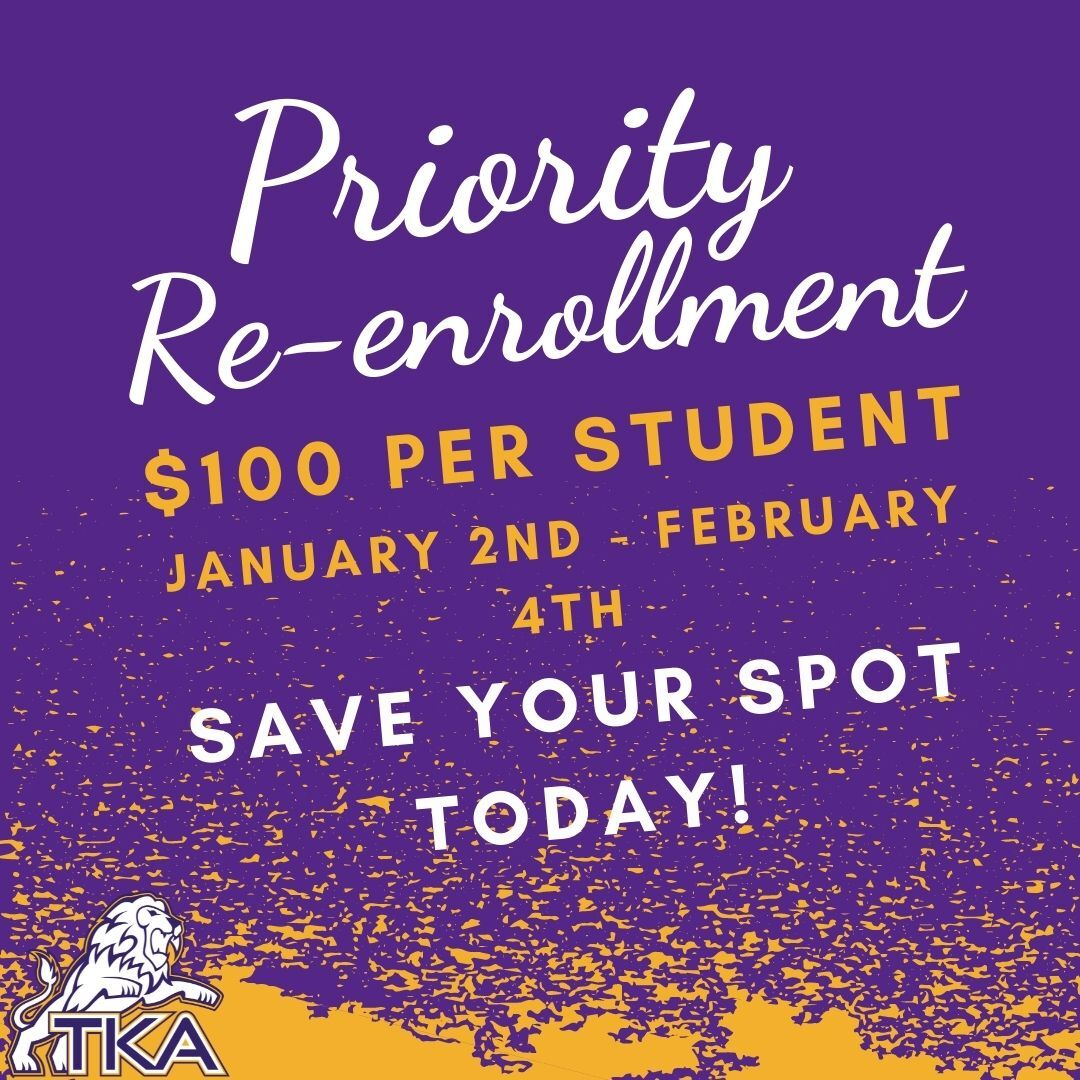# Re-enrollment for 2022-2023

Re-enrollment packets are live in the FACTS Family Portal!

Priority re-enrollment fee of \$100 per student (non-refundable, guarantees your child’s spot) will be from January 2nd – February 4th.

Re-enrollment packets submitted after February 4th will carry a fee of \$300 per student.

The following are to be submitted:
• Re-enrollment form (through FACTS)
• Payment (any of the following options are acceptable):
1. Cash (submitted to either grade level office)
2. Check (submitted to either grade level office)
3. Payment through FACTS account (families will receive more information regarding how to pay this way. This allows for the use of a card or bank draft as a payment option).

If you have a sibling that will be new to TKA for the 2022-2023 school year, please contact Lori Campbell to begin the application process.

Lori Campbell
(865) 573-8321 option 1

 table div table+table+table+table+table+table div table{width:100%;padding:0}table div table+table+table+table+table+table div table img{width:96.23%;padding:0;float:none}table div table+table+table+table+table+table div table td{width:100%;padding:0 1.88% 18px}/* styles */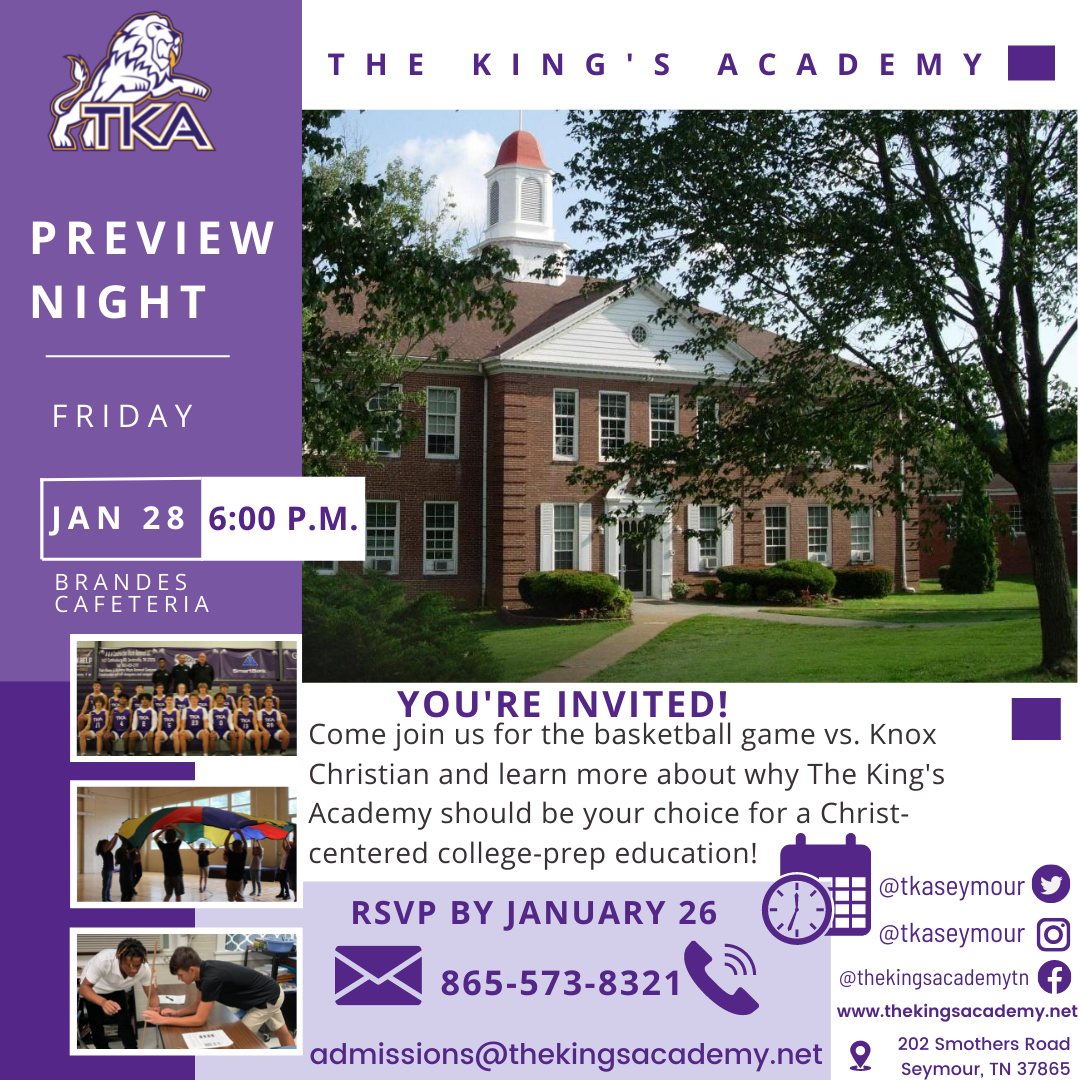We are excited to announce that we have rescheduled our Winter Preview Night for this Friday, January 28, at 6:00. The program for the evening will include information about our school shared by our Headmaster and administrative staff, along with complimentary tickets to our varsity basketball games and some concessions bucks for the event.

We welcome any new families who would like to know more about The King's Academy to attend. If you previously signed up to attend, please confirm your reservation by contacting Lori Campbell by email at lcampbell@thekingsacademy.net. If you have not filled out your RSVP information, click here to RSVP by January 26!

 table div table+table+table+table+table+table+table+table div table{width:100%;padding:0}table div table+table+table+table+table+table+table+table div table img{width:96.23%;padding:0;float:none}table div table+table+table+table+table+table+table+table div table td{width:100%;padding:0 1.88% 18px}/* styles */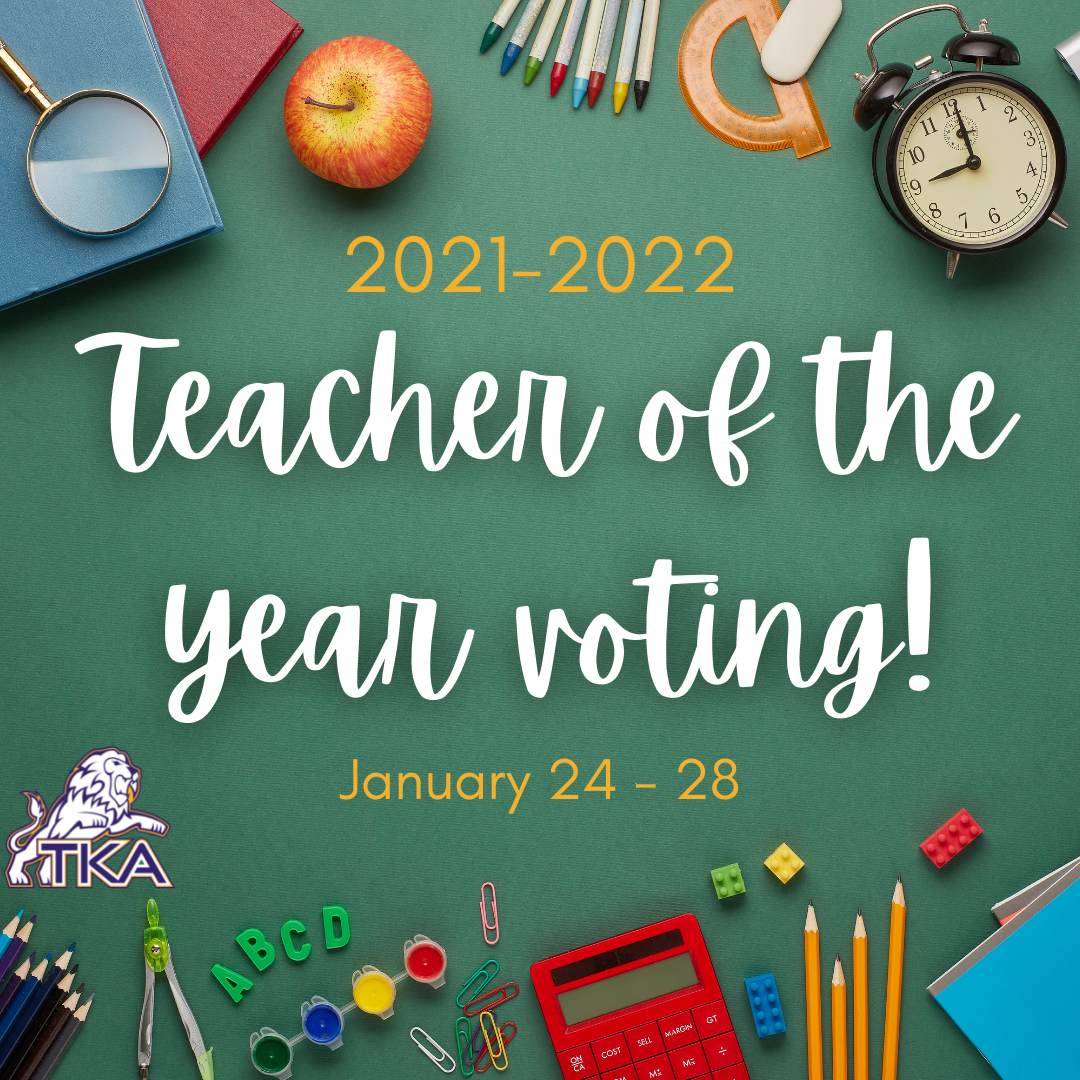# Teacher of the Year Voting

Teacher of the Year Nominations are in, and it is time to vote! Voting will take place from January 24 - January 28 at 3:00 p.m. All voting will be done electronically through this link. Students, parents, teachers and staff are welcome to vote! One vote per person please. Parents, please help younger age students vote at home as they will not be voting at school.

 table div table+table+table+table+table+table+table+table+table+table div table{width:100%;padding:0}table div table+table+table+table+table+table+table+table+table+table div table img{width:96.23%;padding:0;float:none}table div table+table+table+table+table+table+table+table+table+table div table td{width:100%;padding:0 1.88% 18px}/* styles */# Love Gala

You're invited to the first annual Love Gala hosted by The King's Academy PTF. The Love Gala will be on February 12, 2022, at Johnson University in Knoxville, TN. Plan on joining us for a night of fellowship, an exciting auction with great items, and a time to honor our alumni, teachers, and those who have left a legacy at TKA. All funds raised will help us love on our teachers, students and facilities.

Each ticket purchased for the event will have an opportunity to win FREE tuition for one student for the 2022-23 academic year. Additional tuition remission opportunity tickets are available for purchase at the door for \$25 per opportunity. All opportunity drawing tickets are Non-Transferable. Only the student drawn is eligible for tuition remission. Must be present to receive tuition remission.To purchase tickets and RSVP for the event, scan this QR Code.

 table div table+table+table+table+table+table+table+table+table+table+table+table+table div table{width:100%;padding:0}table div table+table+table+table+table+table+table+table+table+table+table+table+table div table img{width:96.23%;padding:0;float:none}table div table+table+table+table+table+table+table+table+table+table+table+table+table div table td{width:100%;padding:0 1.88% 18px}/* styles */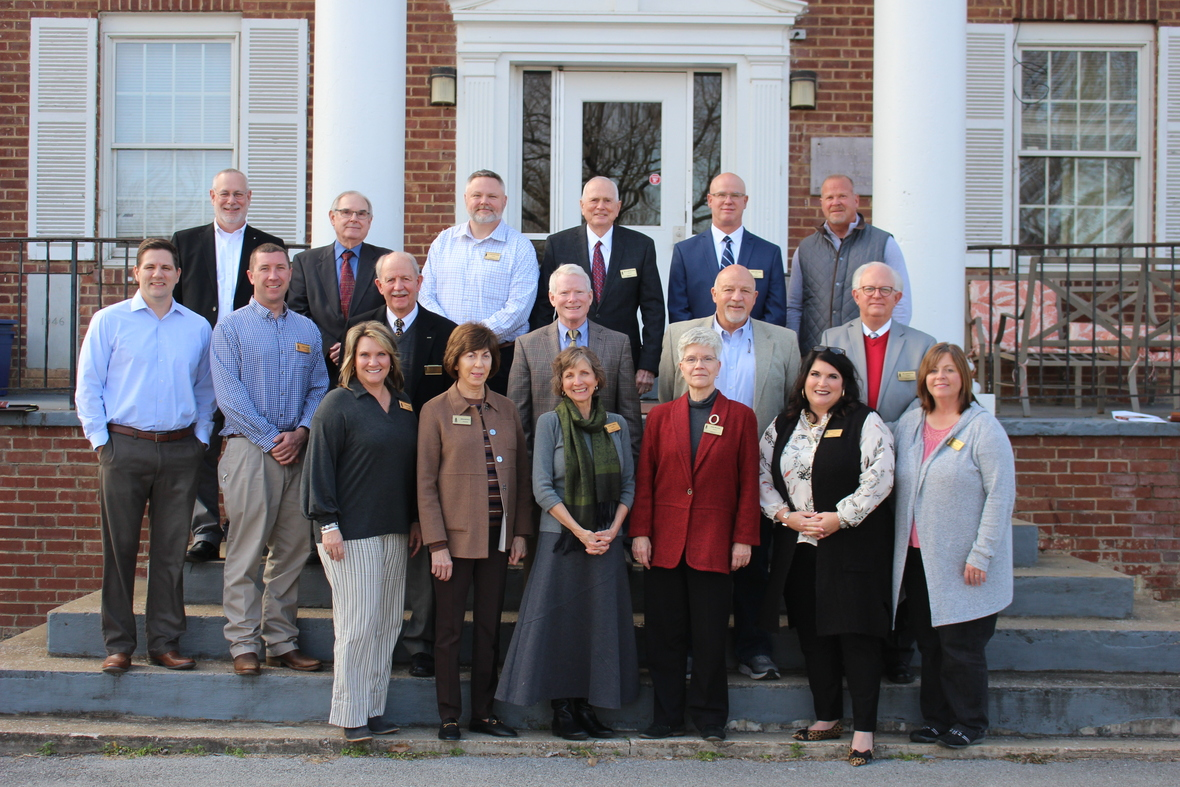# 2022 Board of Trustees

On Tuesday, January 25, our Board of Trustees had their first meeting of the 2022 year. We are thankful for these ladies and gentleman and all they do to help The King's Academy.

The 2022 Board Members pictured here from left to right are as follows:
(bottom row) Mrs. Tiffany Webb, Mrs. Brenda Burchfield, Mrs. Christy Wood, Ms. Jane Wilson (Chairperson), Mrs. Wendy Atchley, Mrs. Julie Knight. (Middle row) Mr. Marshall Stanger, Mr. Daniel Finchum, Mr. Richard Wallace, Mr. Ed Flannigan, Mr. Roger Robinson, Dr. John Nixon. (top row) Mr. Ray "Buddy" Fain, Mr. Joe Van Hook, Dr. Christopher Harding, Dr. Carter Davis, Mr. Drew Smothers, and Mr. Bryan Delius (vice-chairperson).

*Trustees not pictured are: Rev. Gabe Brown, Mr. Omar Cox, and Mr. Kevin Keck.

 table div table+table+table+table+table+table+table+table+table+table+table+table+table+table+table div table{width:100%;padding:0}table div table+table+table+table+table+table+table+table+table+table+table+table+table+table+table div table img{width:96.23%;padding:0;float:none}table div table+table+table+table+table+table+table+table+table+table+table+table+table+table+table div table td{width:100%;padding:0 1.88% 18px}/* styles */Join us this Saturday, January 29th from 4:30 p.m. - 8:00 p.m. for our 8th grade basketball night! 8th graders will be recognized around 6:30-6:45 prior to the all star game.

Gate Fee is \$5 adults and students/kids are free. Concessions will be available for purchase.

 table div table+table+table+table+table+table+table+table+table+table+table+table+table+table+table+table+table div table{width:100%;padding:0}table div table+table+table+table+table+table+table+table+table+table+table+table+table+table+table+table+table div table img{width:96.23%;padding:0;float:none}table div table+table+table+table+table+table+table+table+table+table+table+table+table+table+table+table+table div table td{width:100%;padding:0 1.88% 18px}/* styles */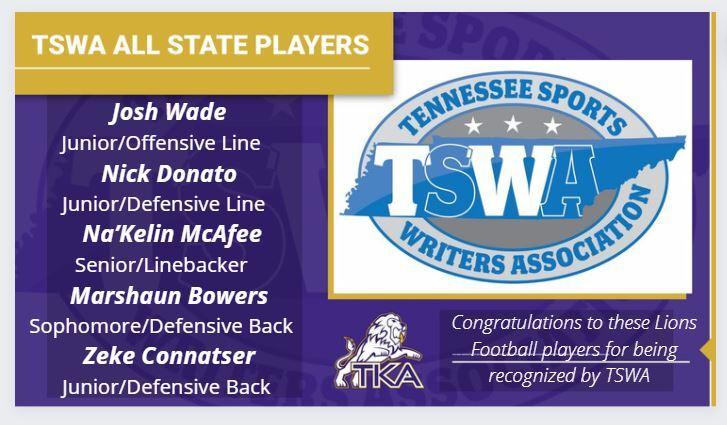# TSWA All State Players

Congratulations to our TKA Lions football players for their recognition by TSWA! We are proud of all of these athletes.

 table div table+table+table+table+table+table+table+table+table+table+table+table+table+table+table+table+table+table+table div table{width:100%;padding:0}table div table+table+table+table+table+table+table+table+table+table+table+table+table+table+table+table+table+table+table div table img{width:96.23%;padding:0;float:none}table div table+table+table+table+table+table+table+table+table+table+table+table+table+table+table+table+table+table+table div table td{width:100%;padding:0 1.88% 18px}/* styles */# Upcoming Spring Dress Up Days

Mark your calendars! Jr. Beta will be hosting dress up days each month to continue to partner with and raise funds for the Isaiah 117 House as we continue to Love Where We Live.
For the donation of \$1.00 (or more) per dress up day, students can wear this fun attire. Please remember that all attire must adhere to dress code.

 table div table+table+table+table+table+table+table+table+table+table+table+table+table+table+table+table+table+table+table+table+table div table{width:100%;padding:0}table div table+table+table+table+table+table+table+table+table+table+table+table+table+table+table+table+table+table+table+table+table div table img{width:96.23%;padding:0;float:none}table div table+table+table+table+table+table+table+table+table+table+table+table+table+table+table+table+table+table+table+table+table div table td{width:100%;padding:0 1.88% 18px}/* styles */# Elementary STEAM Week 2022

This week is our 2nd Annual STEAM (Science, Technology, Engineering, Arts, Math) Week for our Elementary students! Students throughout the different grade levels will participate in workshops from Three Phase Innovations, KUB, Amazon, Preschool STEAM, and MUSE. During this week students have access to our very own STEAM Store (select hours available) to purchase fun science items (pencils, notepads, folders, erasers, and more) along with a variety of snack items for the cost of \$1 each.

For the fun finale on Friday, students will have the opportunity to duct tape Ms. Sullivan to the wall for \$1 per strip.

Check out some of the excitement that has been happening for STEAM week thus far!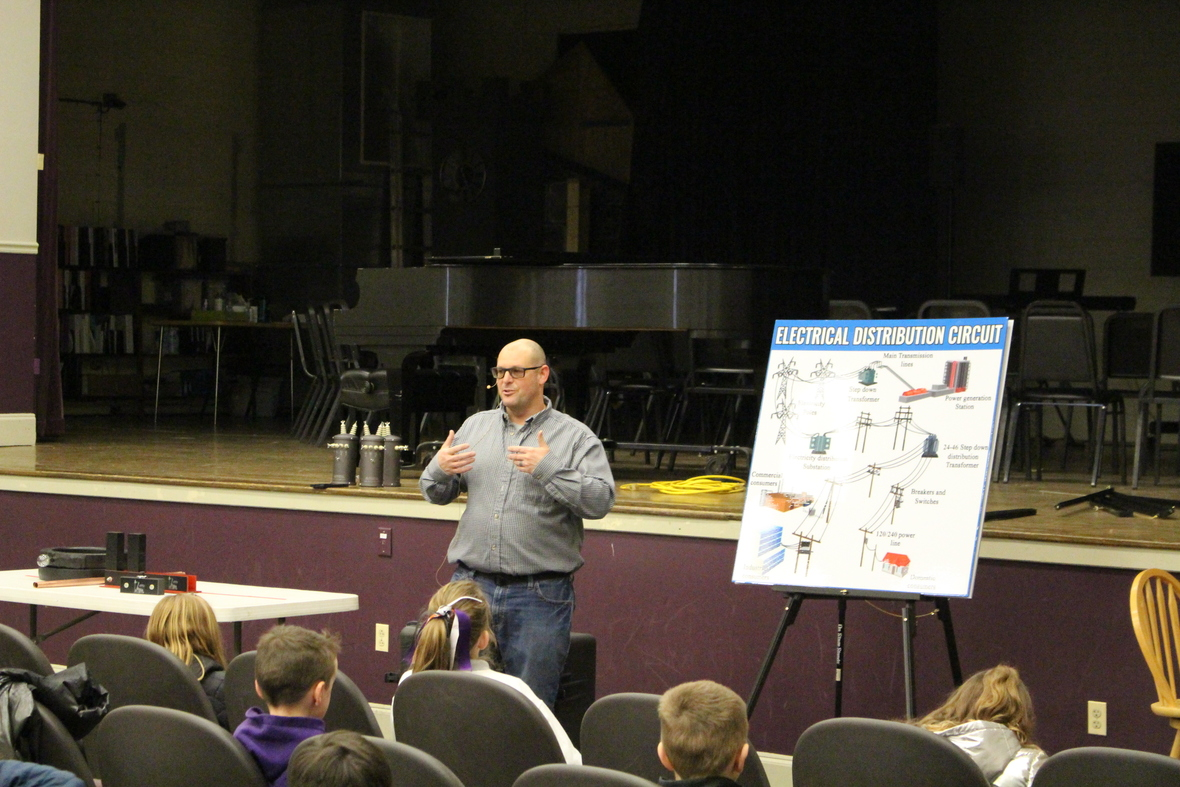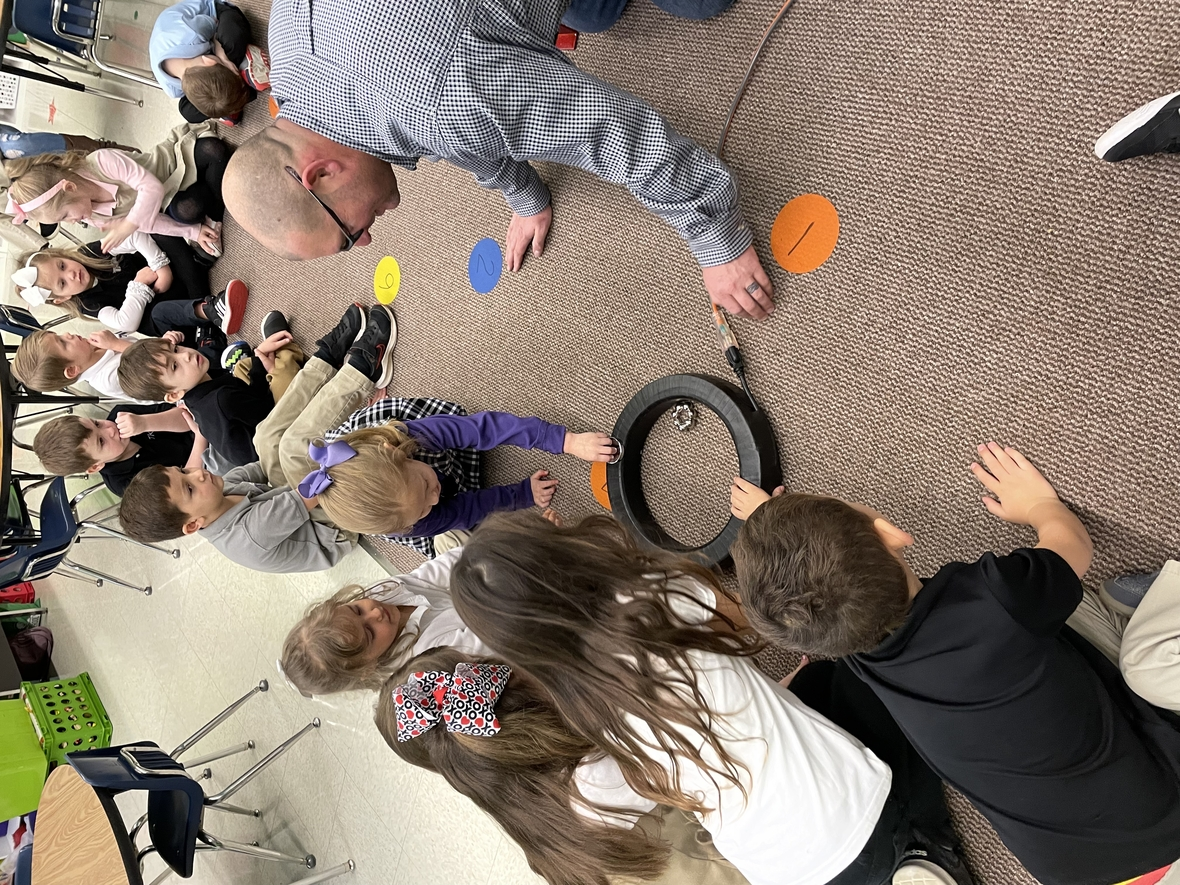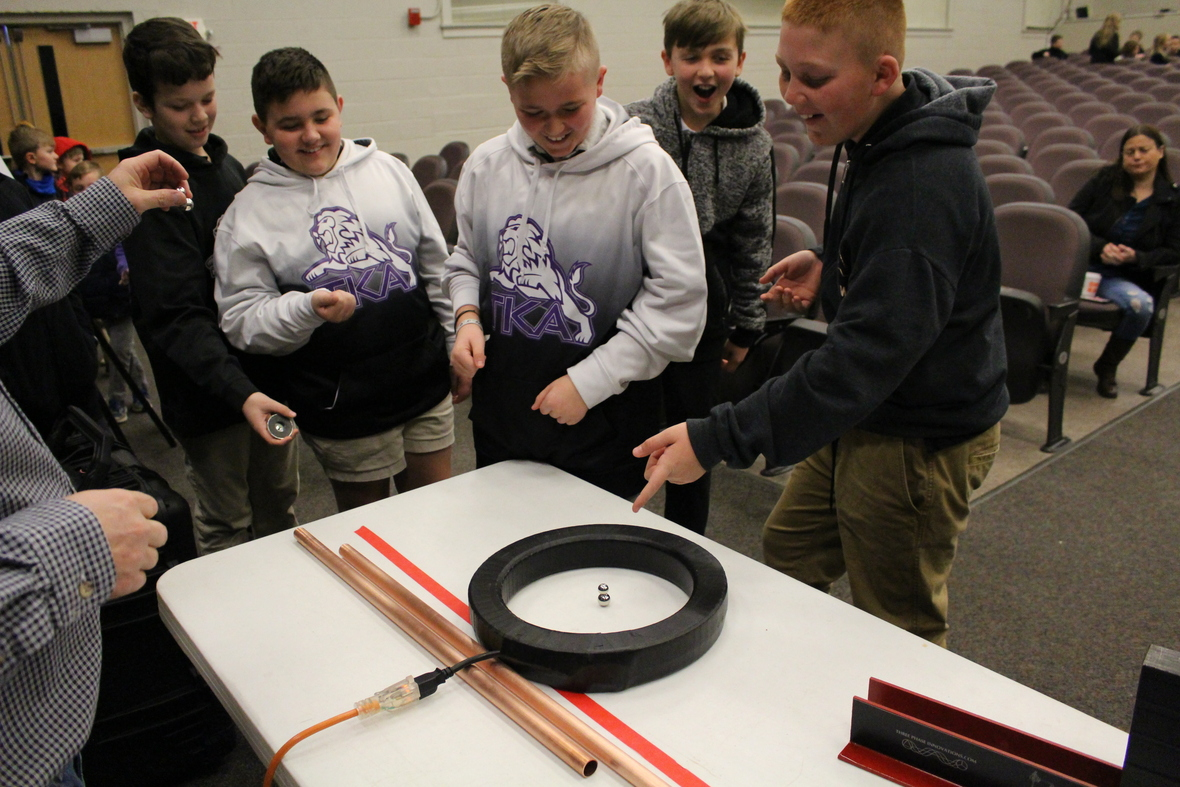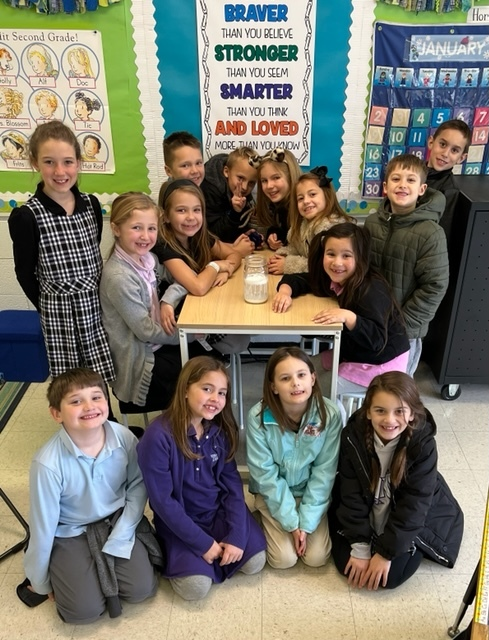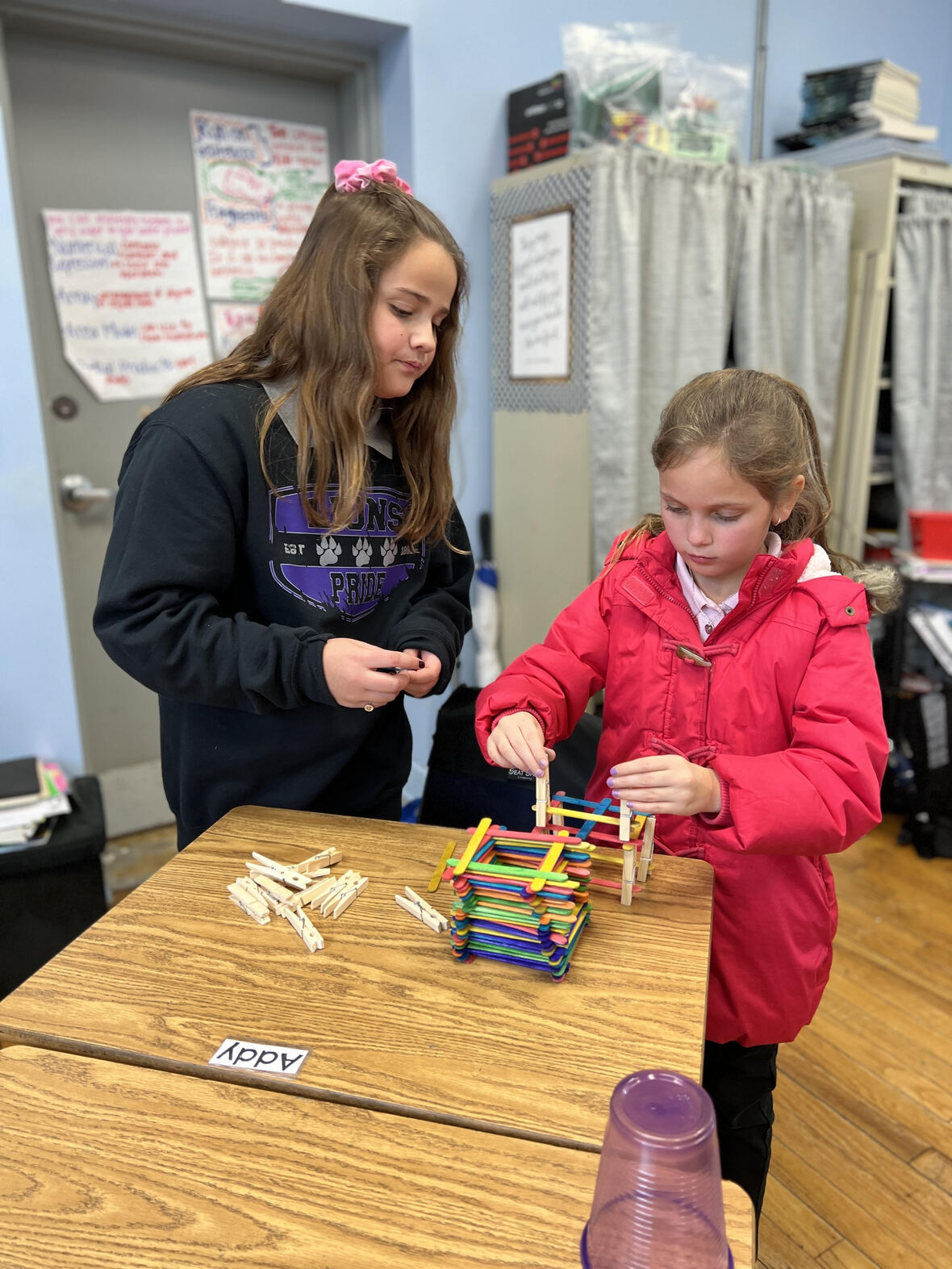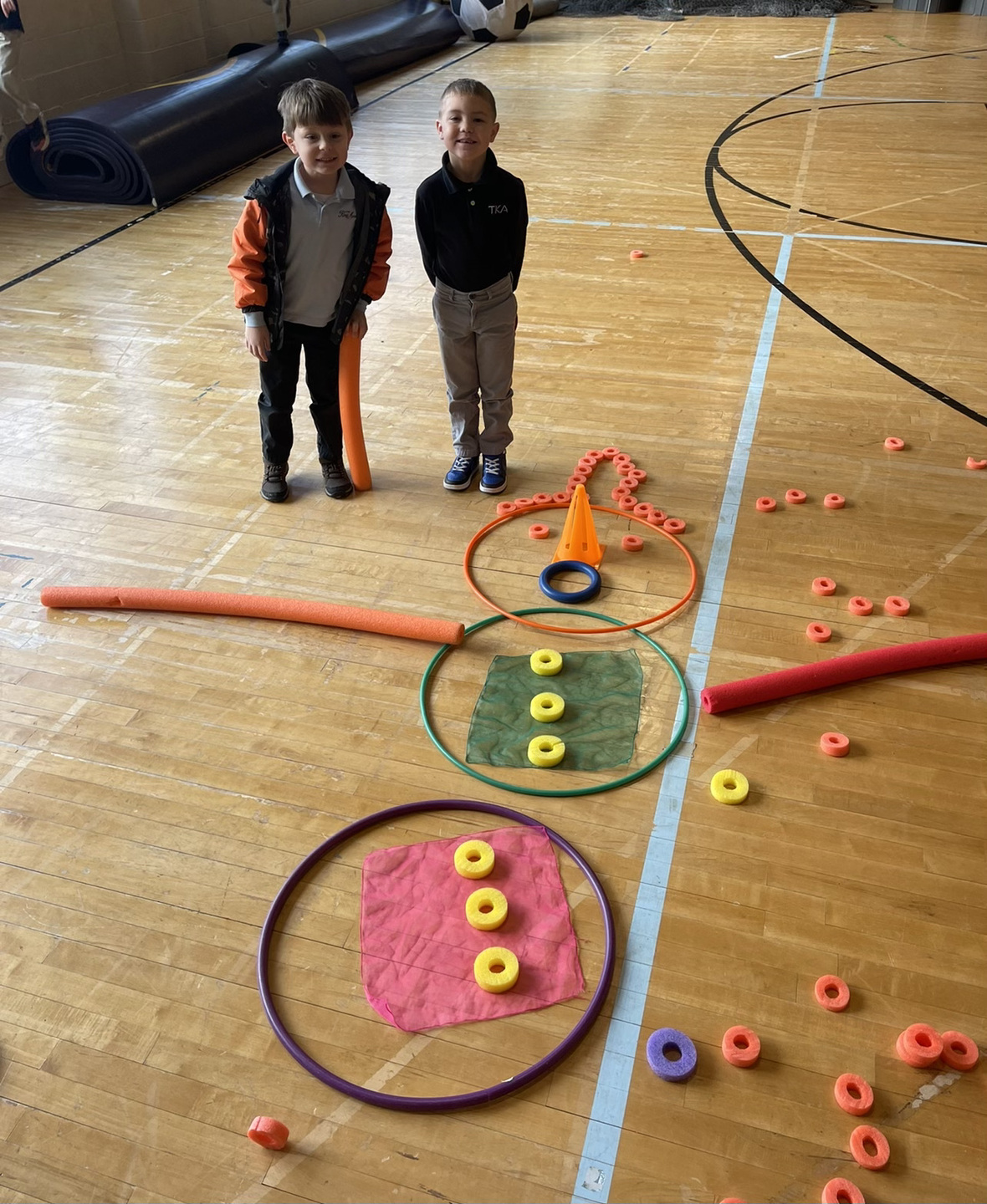table div table+table+table+table+table+table+table+table+table+table+table+table+table+table+table+table+table+table+table+table+table+table+table+table+table div table{width:100%;padding:0}table div table+table+table+table+table+table+table+table+table+table+table+table+table+table+table+table+table+table+table+table+table+table+table+table+table div table img{width:96.23%;padding:0;float:none}table div table+table+table+table+table+table+table+table+table+table+table+table+table+table+table+table+table+table+table+table+table+table+table+table+table div table td{width:100%;padding:0 1.88% 18px}/* styles */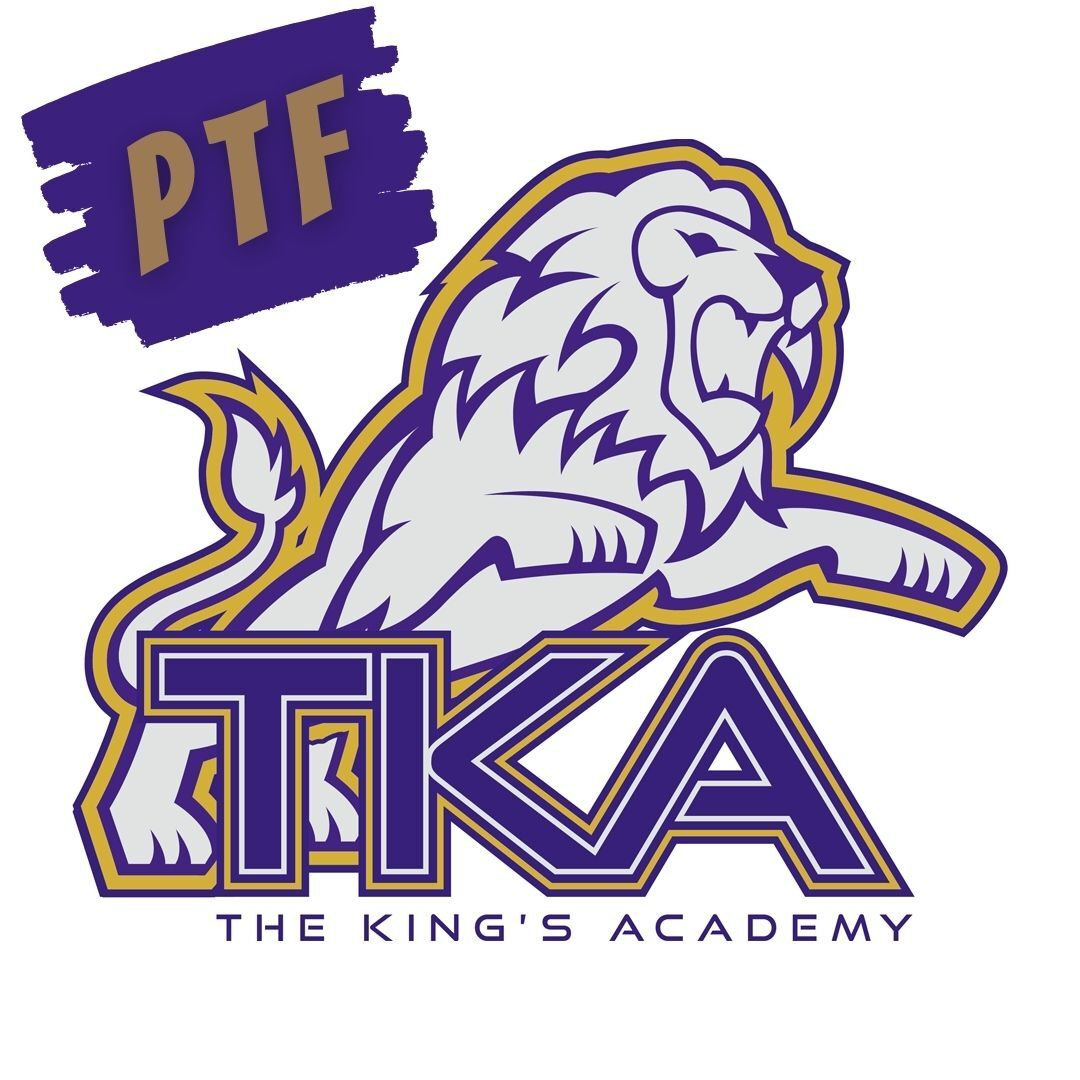# PTF

The next PTF meeting will be Thursday, February 17, from 3:15 PM-4:05 PM in Brandes.

Childcare will be provided throughout the aftercare program free of charge until 4:15 PM for the meeting.

If you haven't joined PTF, no worries, come anyway! Membership forms will be available at the meeting. The fee to join is \$25 per family.

We look forward to having you as part of our PTF this year!

 table div table+table+table+table+table+table+table+table+table+table+table+table+table+table+table+table+table+table+table+table+table+table+table+table+table+table+table div table{width:100%;padding:0}table div table+table+table+table+table+table+table+table+table+table+table+table+table+table+table+table+table+table+table+table+table+table+table+table+table+table+table div table img{width:96.23%;padding:0;float:none}table div table+table+table+table+table+table+table+table+table+table+table+table+table+table+table+table+table+table+table+table+table+table+table+table+table+table+table div table td{width:100%;padding:0 1.88% 18px}/* styles */WHO: PreK3-5th grade students and their Dad (Grandfather, Uncle, or other important male figure)

WHEN: Saturday, February 19, from 6 p.m. to 8 p.m.

WHERE: Sevierville Civic Center (200 Gary R. Wade Blvd)

COST: \$90 per couple/\$45 per additional child (sorry, no refunds) The price includes Dinner and Photo.

For any questions regarding this event, please email Summer Delius at summerdelius@gmail.com.

 table div table+table+table+table+table+table+table+table+table+table+table+table+table+table+table+table+table+table+table+table+table+table+table+table+table+table+table+table+table div table{width:100%;padding:0}table div table+table+table+table+table+table+table+table+table+table+table+table+table+table+table+table+table+table+table+table+table+table+table+table+table+table+table+table+table div table img{width:96.23%;padding:0;float:none}table div table+table+table+table+table+table+table+table+table+table+table+table+table+table+table+table+table+table+table+table+table+table+table+table+table+table+table+table+table div table td{width:100%;padding:0 1.88% 18px}/* styles */# Save The Date

TKA will be hosting the “Forged Parent Conference” on March 5th in Woody Auditorium. “Forged” will be a morning of biblical-based parenting seminars focused on encouraging parents that are raising students in a post-Christian society. We are blessed to have local ministers from our community coming to share on topics involving discipleship, maneuvering technology in today’s digital society, and student identity in an ever-changing environment. This event is free and will be a great opportunity to link arms with other parents as we focus on what the Bible says about the roles and responsibilities associated with raising children. Join us from 9:00 – 12:00 on Saturday, March 5th for “Forged!”

 table div table+table+table+table+table+table+table+table+table+table+table+table+table+table+table+table+table+table+table+table+table+table+table+table+table+table+table+table+table+table+table div table{width:100%;padding:0}table div table+table+table+table+table+table+table+table+table+table+table+table+table+table+table+table+table+table+table+table+table+table+table+table+table+table+table+table+table+table+table div table img{width:96.23%;padding:0;float:none}table div table+table+table+table+table+table+table+table+table+table+table+table+table+table+table+table+table+table+table+table+table+table+table+table+table+table+table+table+table+table+table div table td{width:100%;padding:0 1.88% 18px}/* styles */# Upcoming Weekly Sporting Events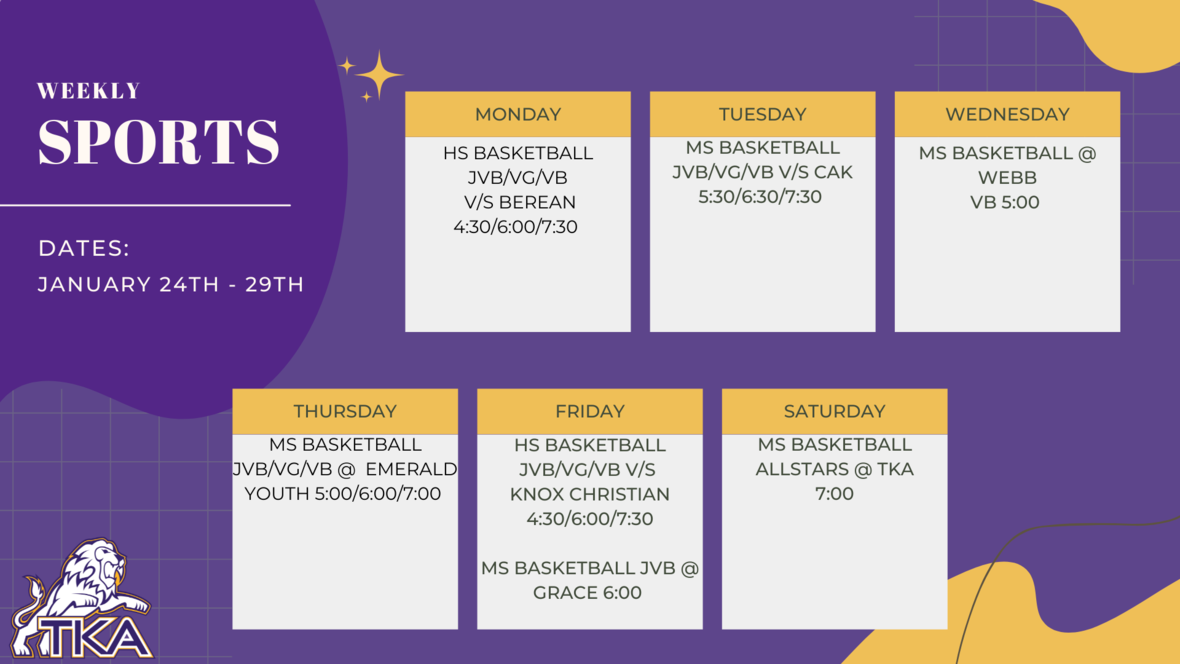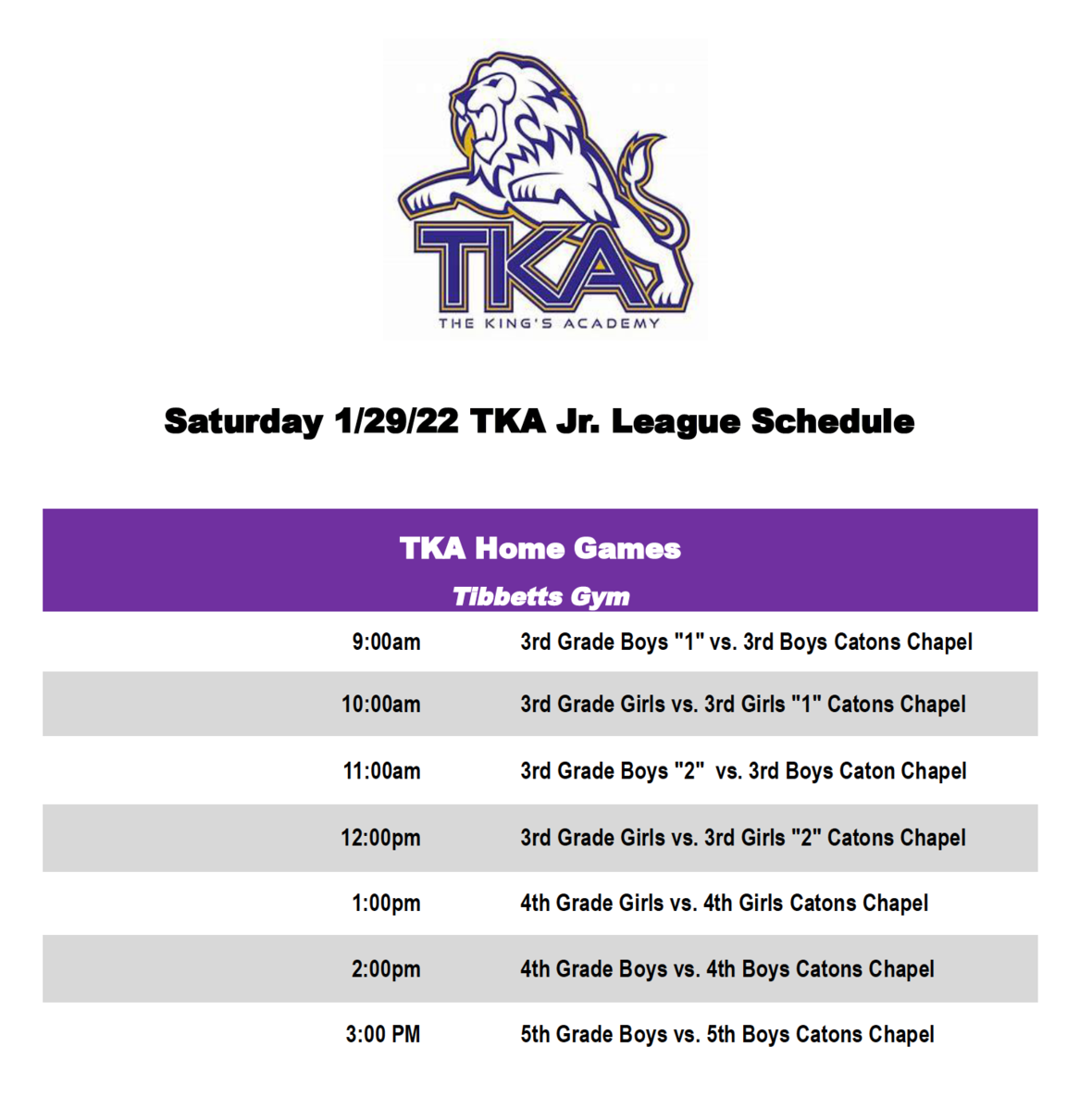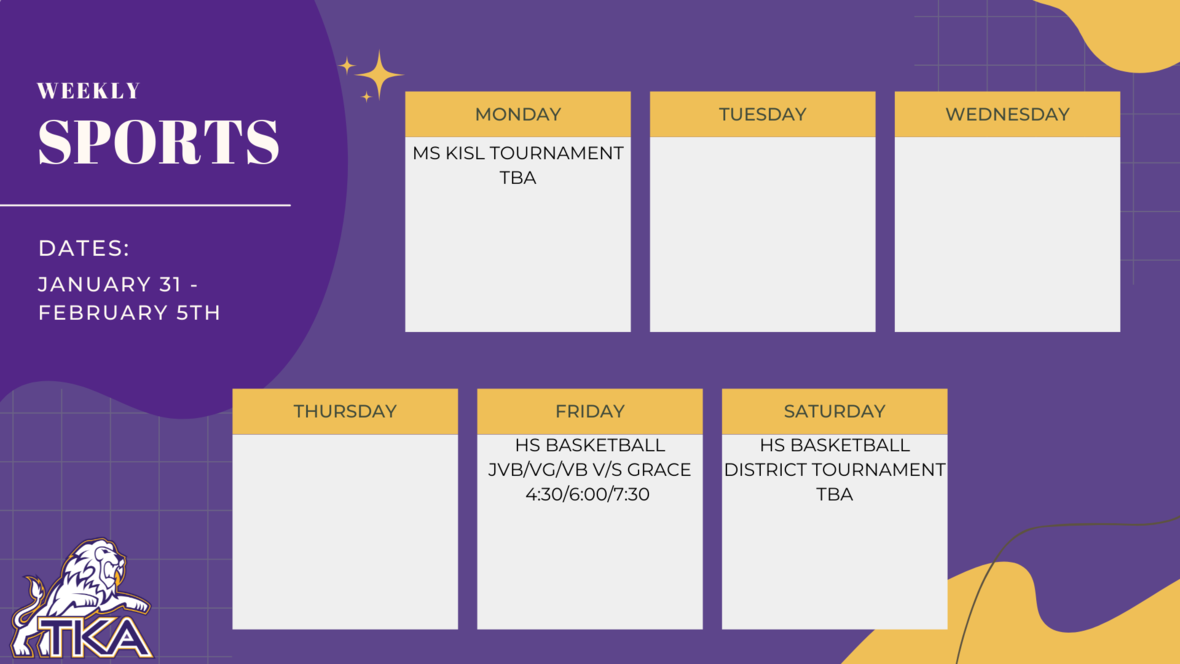table div table+table+table+table+table+table+table+table+table+table+table+table+table+table+table+table+table+table+table+table+table+table+table+table+table+table+table+table+table+table+table+table+table+table+table+table div table{width:100%;padding:0}table div table+table+table+table+table+table+table+table+table+table+table+table+table+table+table+table+table+table+table+table+table+table+table+table+table+table+table+table+table+table+table+table+table+table+table+table div table img{width:96.23%;padding:0;float:none}table div table+table+table+table+table+table+table+table+table+table+table+table+table+table+table+table+table+table+table+table+table+table+table+table+table+table+table+table+table+table+table+table+table+table+table+table div table td{width:100%;padding:0 1.88% 18px}/* styles */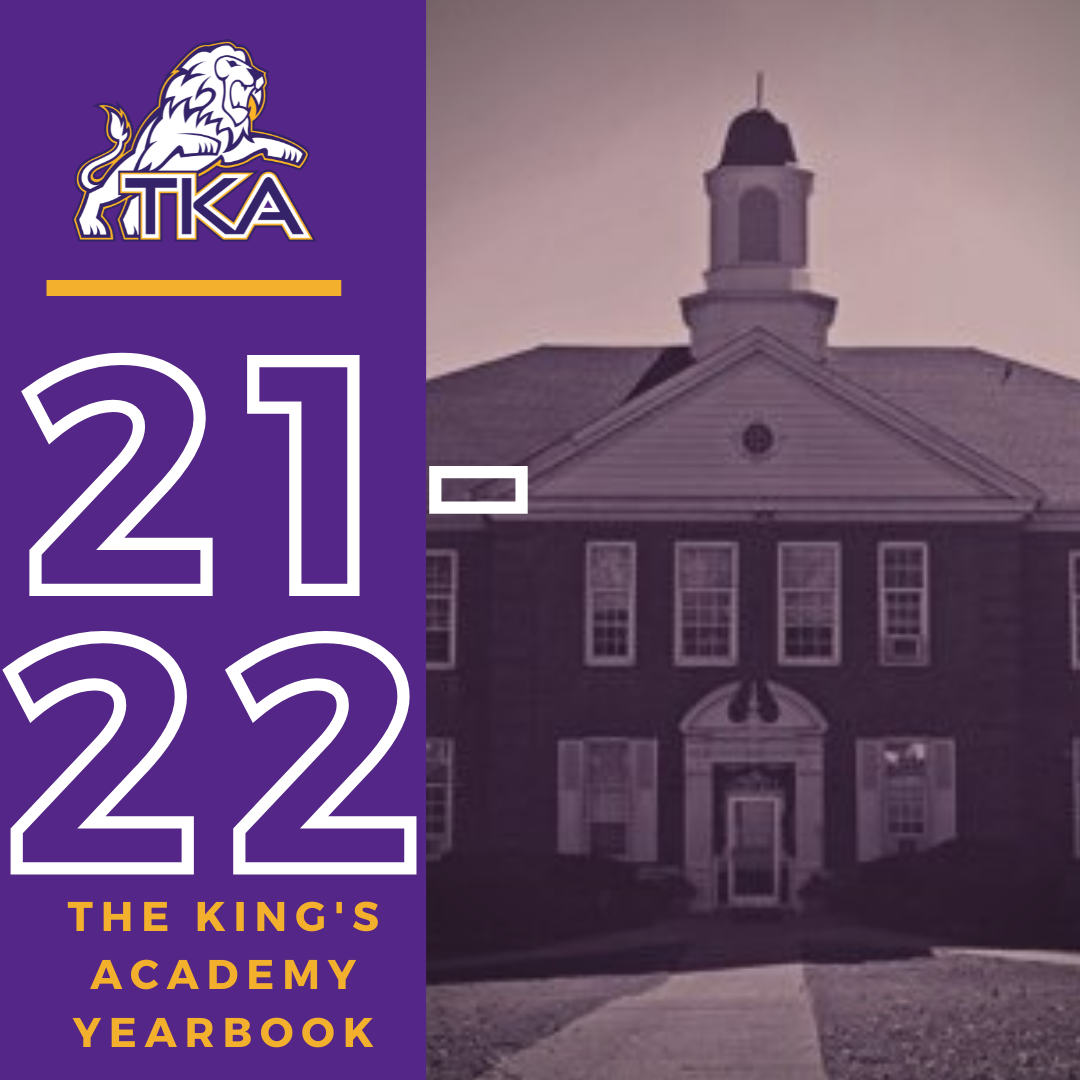# 2021-2022 Yearbook

The price for the 2021-2022 TKA yearbook is \$55. All grades, K3-12 will have the same yearbook. The slight price increase is because we are only going to have one larger all-school book.

All yearbook orders can be placed online--here is the link.

Senior Tributes and Business ads are available for purchase at the bottom of the link listed here.

For any questions regarding yearbooks, email Mr. Josh Smith at jsmith@thekingsacademy.net.

 table div table+table+table+table+table+table+table+table+table+table+table+table+table+table+table+table+table+table+table+table+table+table+table+table+table+table+table+table+table+table+table+table+table+table+table+table+table+table div table{width:100%;padding:0}table div table+table+table+table+table+table+table+table+table+table+table+table+table+table+table+table+table+table+table+table+table+table+table+table+table+table+table+table+table+table+table+table+table+table+table+table+table+table div table img{width:96.23%;padding:0;float:none}table div table+table+table+table+table+table+table+table+table+table+table+table+table+table+table+table+table+table+table+table+table+table+table+table+table+table+table+table+table+table+table+table+table+table+table+table+table+table div table td{width:100%;padding:0 1.88% 18px}/* styles *//* styles */
 table div table+table+table+table+table+table+table+table+table+table+table+table+table+table+table+table+table+table+table+table+table+table+table+table+table+table+table+table+table+table+table+table+table+table+table+table+table+table+table+table+table div table{width:100%;padding:0}table div table+table+table+table+table+table+table+table+table+table+table+table+table+table+table+table+table+table+table+table+table+table+table+table+table+table+table+table+table+table+table+table+table+table+table+table+table+table+table+table+table div table img{width:96.23%;padding:0;float:none}table div table+table+table+table+table+table+table+table+table+table+table+table+table+table+table+table+table+table+table+table+table+table+table+table+table+table+table+table+table+table+table+table+table+table+table+table+table+table+table+table+table div table td{width:100%;padding:0 1.88% 18px}/* styles */table div table+table+table+table+table+table+table+table+table+table+table+table+table+table+table+table+table+table+table+table+table+table+table+table+table+table+table+table+table+table+table+table+table+table+table+table+table+table+table+table+table+table+table div table{width:100%;padding:0}table div table+table+table+table+table+table+table+table+table+table+table+table+table+table+table+table+table+table+table+table+table+table+table+table+table+table+table+table+table+table+table+table+table+table+table+table+table+table+table+table+table+table+table div table img{width:96.23%;padding:0;float:none}table div table+table+table+table+table+table+table+table+table+table+table+table+table+table+table+table+table+table+table+table+table+table+table+table+table+table+table+table+table+table+table+table+table+table+table+table+table+table+table+table+table+table+table div table td{width:100%;padding:0 1.88% 18px}/* styles */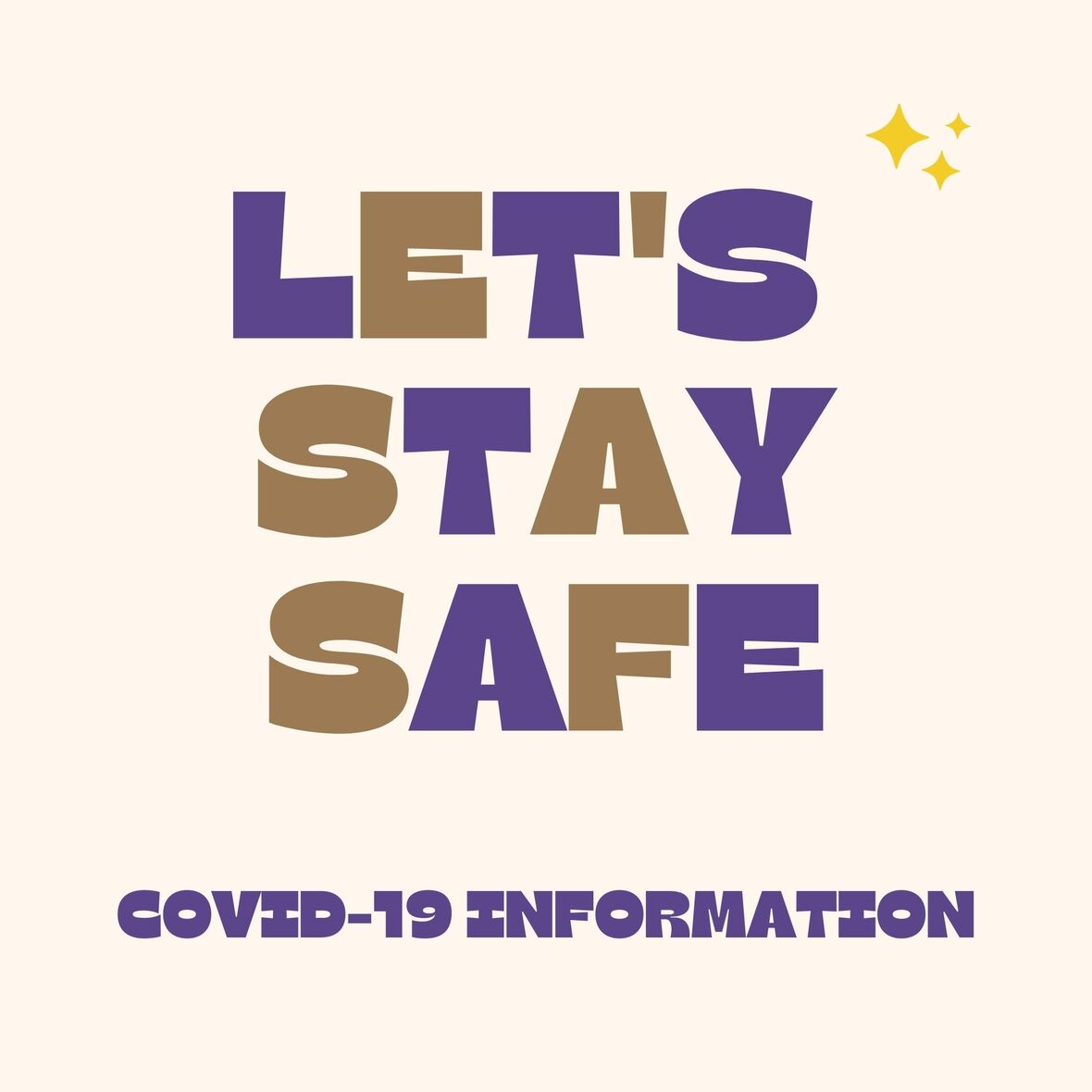# Covid-19 Procedures

While we are excited to offer in-person education, we must maintain a safe learning environment for all students, faculty/staff, and additional stakeholders.

To do so, we will be working closely with the Sevier County Health Department regarding Covid-19 protocols.

 table div table+table+table+table+table+table+table+table+table+table+table+table+table+table+table+table+table+table+table+table+table+table+table+table+table+table+table+table+table+table+table+table+table+table+table+table+table+table+table+table+table+table+table+table+table div table{width:100%;padding:0}table div table+table+table+table+table+table+table+table+table+table+table+table+table+table+table+table+table+table+table+table+table+table+table+table+table+table+table+table+table+table+table+table+table+table+table+table+table+table+table+table+table+table+table+table+table div table img{width:96.23%;padding:0;float:none}table div table+table+table+table+table+table+table+table+table+table+table+table+table+table+table+table+table+table+table+table+table+table+table+table+table+table+table+table+table+table+table+table+table+table+table+table+table+table+table+table+table+table+table+table+table div table td{width:100%;padding:0 1.88% 18px}/* styles */table div table+table+table+table+table+table+table+table+table+table+table+table+table+table+table+table+table+table+table+table+table+table+table+table+table+table+table+table+table+table+table+table+table+table+table+table+table+table+table+table+table+table+table+table+table+table+table div table{width:100%;padding:0}table div table+table+table+table+table+table+table+table+table+table+table+table+table+table+table+table+table+table+table+table+table+table+table+table+table+table+table+table+table+table+table+table+table+table+table+table+table+table+table+table+table+table+table+table+table+table+table div table img{width:96.23%;padding:0;float:none}table div table+table+table+table+table+table+table+table+table+table+table+table+table+table+table+table+table+table+table+table+table+table+table+table+table+table+table+table+table+table+table+table+table+table+table+table+table+table+table+table+table+table+table+table+table+table+table div table td{width:100%;padding:0 1.88% 18px}/* styles */